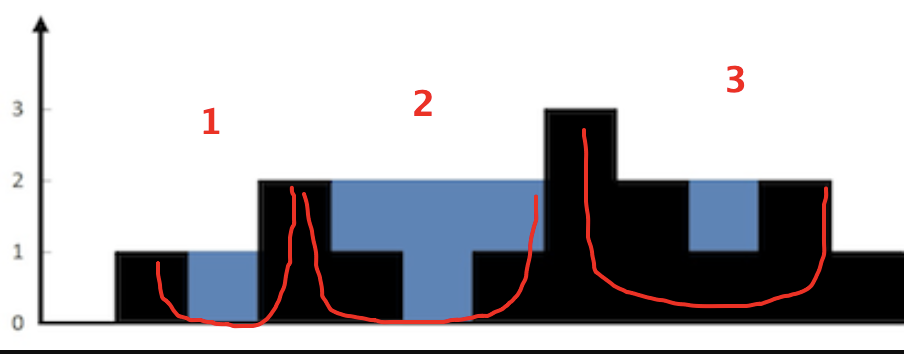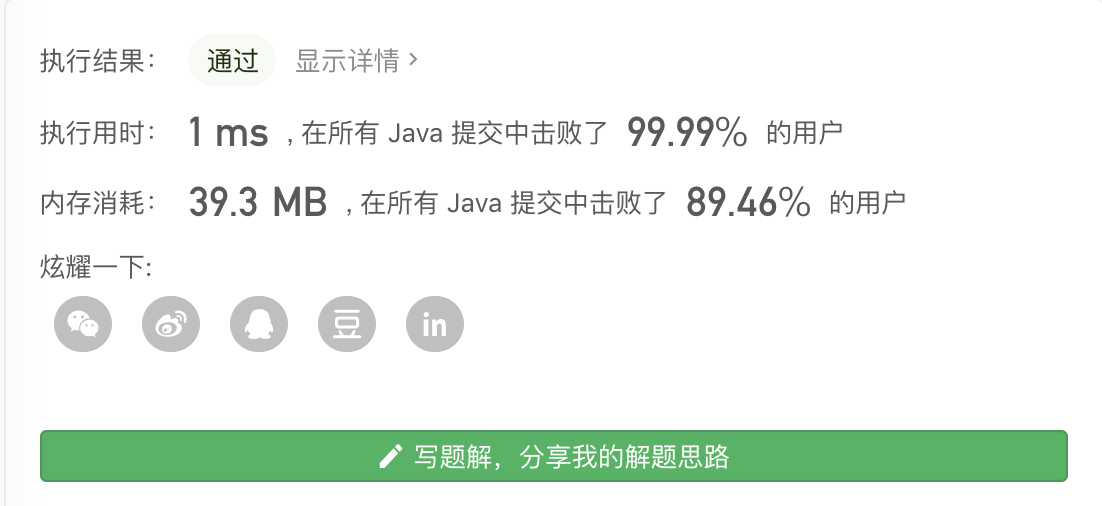# 42. 接雨水

``````class Solution {

public int trap(int[] height) {
int sum = 0;
int left = getFirstLeft(height);
int right;

while (left < height.length-1) {
right = getRight(height, left);
//统计杯子中的水量
sum = sum + (right - left-1) * Math.min(height[left], height[right]);
//去掉杯底非水的部分
for (int i = left + 1; i < right; i++) {
sum = sum - height[i];
}
//找下一个杯子，此时新杯子的左杯壁为当前杯子的右杯壁
left = right;
}
return sum;
}

/**
* 获取第一个左杯壁的位置
* @param height
* @return
*/
private int getFirstLeft(int[] height){
for (int i = 0; i < height.length; i++) {
if (height[i] >0) {
//只要有高度，就算
return i;
}
}
return height.length;
}

/**
* 获取右杯壁的位置
* @param height
* @param left
* @return
*/
private int getRight(int[] height, int left) {
//默认右杯壁位置为整个数组的最右边
int right = height.length-1;
for (int i = left+1; i < height.length; i++) {
//从左杯壁+1位置开始找
if (height[i] >= height[left]) {
//第一个高度大于等于左杯壁的就是右杯壁
return i;
} else if (height[i] > height[right]) {
//如果上述条件找不到，即当前左杯壁已经是最高，则寻找右边元素中最高的值做右杯壁
right = i;
}
}
return right;
}

}
``````